# RS Aggarwal Solutions Chapter 13 Construction Exercise 13A Class 10 MathsChapter Name RS Aggarwal Chapter 13 Construction Book Name RS Aggarwal Mathematics for Class 10 Other Exercises Exercise 13B Related Study NCERT Solutions for Class 10 Maths

### Exercise 13A Solutions

1. Draw a line segment AB of length 7 cm. Using ruler and compasses, find a point P on AB such that AP/AB = 3/5.

Solution

Steps of Construction:

Step 1: Draw a line segment AB = 7 cm

Step 2: Draw a ray AX, making an acute angle ∠BAX.

Step 3: Along AX, mark 5 points (greater of 3 and 5) A1, A2, A3, A4 and A5 such that

AA1 = A1A2 = A2A3 = A3A4 = A4A5

Step 4: Join A5B.

Step 5: From A3, draw A3P parallel to A5B (draw an angle equal to ∠AA5B), meeting AB in P.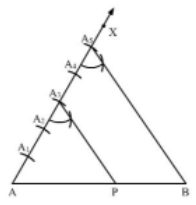Here, P is the point on AB such that AP/PB = 3/2 or AP/AB = 3/5.

2. Draw a line segment of length 7.6 cm and divide it in the ration 5: 8. Measure the two parts.

Solution

Steps of Construction:

Step 1: Draw a line segment AB = 7.6 cm

Step 2: Draw a ray AX, making an acute angle ∠BAX.

Step 3: Along AX, mark (5 + 8 = 13) points A1, A2, A3, A4, A5, A6, A7, A8, A9, A10, A11, A12 and A13 such that

AA1 = A1A2 = A2A3 = A3A4 = A4A5 = A6A7 = A8A9 = A9A10 = A10A11 = A11A12 – A12A13.

Step 4: Join A13B.

Step 5: From A5, draw A5P parallel to A13B (draw an angle equal to ∠AA13B), meeting AB in P.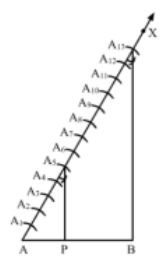Here, P is the point on AB which divides it in the ratio 5 : 8.

∴ Length of AP = 2.9 cm (Approx)

Length of BP = 4.7 cm (Approx)

3. Construct a ΔPQR, in which PQ = 6 cm, QR = 7 cm and PR = -8 cm. Then, construct another triangle whose sides are 4/5 times the corresponding sides of ΔPQR.

Solution

Steps of Construction

Step 1: Draw a line segment QR = 7 cm.

Step 2: With Q as center and radius 6 cm, draw an arc.

Step 3: With R as center and radius 8 cm, draw an arc cutting the previous arc at P

Step 4: Join PQ and PR. Thus, ΔPQR is the required triangle.

Step 5: Below QR, draw an acute angle ∠RQX.

Step 6: Along OX, mark five points R1, R2, R3, R4 and R5 such that

QR1 = R1R2 = R2R3 = R3R4 = R4R5.

Step 7: Join RR5

Step 8: From R4, draw R4R’ ∥ RR5 meeting QR at R’.

Step 9: From R’, draw P’R’ ∥ PR meeting QR at R’.

Here, ΔP’QR’ is the required triangle, each of whose sides are 4/5 times the corresponding sides of ΔPQR.

4. Construct a triangle with sides 5 cm, 6 cm, and 7 cm and then another triangle whose sides are 7/5 of the corresponding sides of the first triangle.

Solution

Steps of construction:

Step 1: Draw a line segment BC = 4 cm.

Step 2: With B as center, draw an angle of 90°.

Step 3: With B as center and radius equal to 3 cm, cut an arc at the right angle and name it A.

Step 4: Join AB and AC.

Thus, ΔABC is obtained.

Step 5: Extend BC to D, such that BD = 7/5.BC = 75(4) cm = 5.6 cm

Step 6: Draw DE ∥ CA, cutting AB produced to E.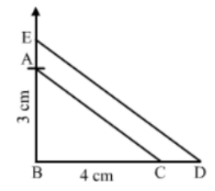Thus, ΔEBD is the required triangle, each of whose sides is 7/5 the corresponding sides of ΔABC.

5. Construct a ΔABC with BC = 7 cm, B = 60° and AB = 6 cm. Construct another triangle whose sides are 3/4 times the corresponding sides of ΔABC.

Solution

Steps of construction

Step 1: Draw a line segment BC = 7 cm

Step 2: At B, draw ∠XBC = 60°.

Step 3: With B as center and radius 6 cm, draw an arc cutting the ray BX at A.

Step 4: Join AC, Thus, ΔABC is the required triangle.

Step 5: Below BC, draw an acute angle ∠YBC.

Step 6: Along BY, mark four points B1, B2, B3 and B4 such that BB1 = B1B2 = B2B3 = B3B4.

Step 7: Join CB4.

Step 8: From B, draw B3C’ ∥ CB4 meeting BC at C’’.

Step 9: From C’, Draw A’C’ ∥ AC meeting AB in A’.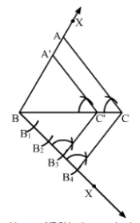Here, ΔA’BC’ is the required triangle whose sides are 3/4 times the corresponding sides of ΔABC.

6. Construct a ΔABC in which AB = 6 cm, A = 30° and AB = 60°, Construct another ΔAB’C’ similar to ΔABC with base AB’ = 8 cm.

Solution

Steps of Construction

Step 1: Draw a line segment AB = 6 cm

Step 2: At A, draw ∠XAB = 30°.

Step 3: At B, draw ∠YBA = 60°. Suppose AX and BY intersect at C.

Thus, ΔABC is the required triangle.

Step 4: Produce AB to B’ such that AB’ = 8 cm

Step 5: From B’, draw B’C’ ∥ BC meeting AX at C’.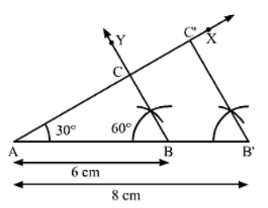Here, AB’C’ is the required triangle similar to ΔABC.

7. Construct a ΔABC in which BC = 8 cm, B = 45° and C = 60°. Construct another triangle similar to ΔABC such that its sides are 3/5 of the corresponding sides of ΔABC.

Solution

Steps of Construction:

Step 1: Draw a line segment BC = 8 cm.

Step 2: At B, draw ∠XBC = 45°.

Step 3: At C, draw ∠YCB = 60°. Suppose BX and CY intersect at A.

Thus, ΔABC is the required triangle.

Step 4: Below BC, draw an acute angle ∠ZBC.

Step 5: Along BZ, mark five points Z1, Z2, Z3, Z4 and Z5 such that

BZ1 = Z1Z2 = Z2Z3 = Z3Z4 = Z4Z5.

Step 6: Join CZ5.

Step 7: From Z3, draw Z3C’ ∥ CZ5 meeting BC at C’.

Step 8: From C’, draw A’C ∥ AC meeting AB in A’.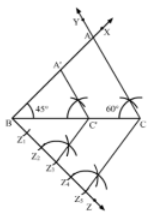Here, ΔA’BC’ is the required triangle whose sides are 3/5 of the corresponding sides of ΔABC.

8. To construct a triangle similar to ΔABC in which BC = 4.5 cm, B = 45° and C = 60°, using a scale factor of 3/7, BC will be divided in the ratio

(a) 3 : 4

(b) 4 : 7

(c) 3 : 10

(d) 3 : 7

Solution

(a) 3 : 4

To construct a triangle similar to ΔABC in which BC = 4.5 cm, ∠B = 45° and ∠C = 60°, using a scale factor of 3/7, BC will be divided in the ratio 3 : 4.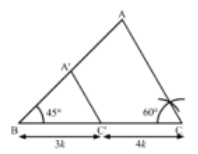Here, ΔABC ΔA’BC’

BC’: C’C = 3 : 4

Or BC’: BC = 3 : 7

Hence, the correct answer is option A.

9. Construct an isosceles triangle whose base is 8 cm and altitude 4 cm and then another triangle whose sides are 1.1/2 times the corresponding sides of the isosceles triangle.

Solution

Steps of Construction:

Step 1: Draw a line segment BC = 8 cm.

Step 2: Draw the perpendicular bisector XY to BC, cutting BC at D.

Step 3: With D as center and radius 4 cm, draw an arc cutting XT at A.

Step 4: Join AB and AC. Thus, an isosceles ΔABC whose base is 8 cm and altitude 4 cm is obtained.

Step 5: Extend BC to E such that BE = 3/2 BC = 3/2 × 8 cm = 12 cm

Step 6: Draw EF ∥ CA, cutting BA produced in F.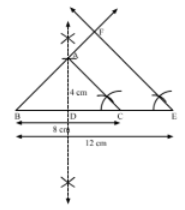Here, ΔBEF is the required triangle similar to ΔABC such that each side of ΔBEF is 1.1/2 (or 3/2) times the corresponding side of ΔABC.

10. Draw a right triangle in which the sides (other than hypotenuse) are of lengths 4 cm and 3 cm, Then, Construct another triangle whose sides are 5/3 times the corresponding sides of the given triangle.

Solution

Steps of Construction

Step 1: Draw a line segment BC = 3 cm.

Step 2: At B, draw ∠XBC = 90°.

Step 3: With B as center and radius 4 cm, draw an arc cutting BX at A.

Step 4: Join AC. Thus, a right ΔABC is obtained.

Step 5: Extend BC to D such that BD = 5/3 BC = 5/3 × 3 cm = 5 cm.

Step 6: Draw a DE ∥ CA, cutting BX in E.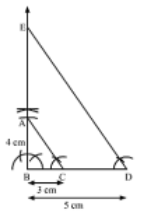Here, ΔBDE is the required triangle similar ΔABC such that each side of ΔBDE is 5/3 times the corresponding side of ΔABC.